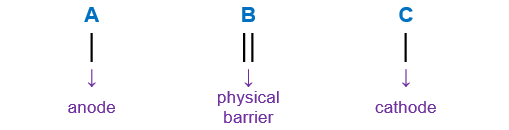# Problem: About how many grams of Sn+2(aq) are formed when 2 A is run through the cell below for 3 hours?Sn+4(aq) I Sn+2(aq) II Pb2+(aq) I Pb(s)1) about 10 grams2) about 20 grams3) about 5 grams4) none, Sn+2(aq) is not formed in the reaction.

###### FREE Expert Solution

We’re being asked to calculate the mass of Sn2+(aq) are formed when 2 A is run through the cell below for 3 hours.

Sn4+(aq) I Sn2+(aq) II Pb2+(aq) I Pb(s)

Let’s first determine how many electrons are involved in the reaction:

When writing a cell notation, we use the following format – “as easy as ABC99% (422 ratings)###### Problem Details

About how many grams of Sn+2(aq) are formed when 2 A is run through the cell below for 3 hours?
Sn+4(aq) I Sn+2(aq) II Pb2+(aq) I Pb(s)